abstractmath.org 2.0
help with abstract math

Revised 2017-04-08      Introduction to this website       website TOC


# UNDERSTANDING MATH: CONCEPT AND COMPUTATION

## Introduction

When mathematicians consider a mathematical object, they are typically interested in two different aspects of it:

### Conceptual: What is it?

I want a conceptual understanding of the object.

• How do I think about it?
• What properties does it have?
• How is it different from other math objects?
• How can I understand it so that I can see possible applications?

### Computational: How do I compute with it?

• How do I find a value of the object (if that makes sense)?
• How to I tell how big it is (in some sense of big)?
• How do I determine in an efficient way what properties it has?

### Proofs

Proofs can have a conceptual side and a computational side too.

• A conceptual proof helps you understand why the statement is true.
• A computational or symbolic proof may not help your understanding, but it will probably be easier to check systematically to see if it is correct, and to automate using some suitable computer program.

#### Conceptual and computational are not well defined ideas

• Conceptual presentations are commonly geometric, but they don't have to be.
• If you understand some technicalities really well, you might call something conceptual that looks like a horrible bunch of symbolic manipulations to someone else.
• Whether something is conceptual or not depends on what concepts you understand!

## Example: An algebraic identity

Here is a simple example that shows the distinction between concept and computation. This identity ${{a}^{2}}-{{b}^{2}}=(a-b)(a+b)$ holds for all real numbers. (In fact it holds in any commutative ring.)

### Computational proof

This proof shows an explicit series of steps that verify the identity using basic laws of algebra: \begin{align*} (a-b)(a+b)&= (a-b)a+(a-b)b \;\;\; \text{distributive law} \\ &= {{a}^{2}}-ba+ab-{{b}^{2}} \;\;\; \text{distributive law applied twice} \\ &= {{a}^{2}}-ab+ab-{{b}^{2}} \;\;\; \text{commutative law} \\ &= {{a}^{2}}+0-{{b}^{2}} \\ &= {{a}^{2}}-{{b}^{2}} \end{align*}

These laws hold in every commutative ring, so the identity holds in every commutative ring.

### Conceptual proof

This diagram shows why the identity is true geometrically (for $b\le a$).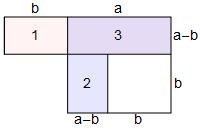Boxes 1 and 2 are congruent, so have the same area. Therefore the area of boxes 1 and 3 is the same as the area of boxes 2 and 3. But the first one has area $(a+b)(a-b)$ and the second one has area $a^2-b^2$, so necessarily $a^2-b^2=(a+b)(a-b)$.

This conceptual proof requires no algebraic laws at all. On the other hand, it is not easy to see how to generalize it to a commutative ring. Think about drawing a picture that shows that${{a}^{2}}-{{b}^{2}}=(a-b)(a+b)$ for all complex numbers $a$ and $b$.

## Example: Property of the ordering of the reals

The idea of "conceptual proof" depends on your experience. If you are not familiar with basic geometric facts, the preceding geometric proof may not be conceptual!

Here is another example that shows how "conceptual" depends on what you know.

### Theorem:

For all real numbers $x$ $y$, and $z$, if $x\le z$, then either $x\le y$ or $y\le z$.

#### Assume that one alternative is false and see what happens

This is a standard trick for proving that "$P$ or $Q$", namely prove directly that if $P$ is false then $Q$ has to be true.

If $x\leq y$ is not true, then it must be true that $y\lt x$. We are given that $x\leq z$, so now we know that $y\lt x$ and $x\leq z$. It follows from that fact that the ordering of the reals is transitive that $y\leq z$.

#### Geometric proof

The number $y$ has to be in one of three intervals in this picture of (part of) the real line. (The left interval goes off to infinity to the left and the right interval goes off to infinity to the right. The middle interval has finite length.)If y is in the left interval or the middle interval, then $y\le z$. If it is in the middle or right interval, then $x\le y$.

#### Logical Proof

• The transitive law says that if $x\gt y$ and $y \gt z$, then $x \gt z$.
• The contrapositive of this statement is: If $x$ is not greater than $z$ then either $x$ is not greater than $y$ or $y$ is not greater than $z$ (this uses a DeMorgan Law).
• But for any real numbers $r$ and $s$, saying that $r$ is not greater than $s$ is the same as saying that $r\le s$.
• So, rewording the contrapositive, we get: if $x\le z$, then either $x\le y$ or $y\le z$, as was to be proved.

If you have some experience with mathematical logic, you might react to the logical proof (as I did) this way:

The statement in the theorem is nothing but the contrapositive of the transitive law!

When I realized that, I felt that I had acquired a new insight, so I consider this to be a conceptual proof. The geometric proof gives you a different conceptual insight.

## Example: Property of the greatest common divisor

### Some basic facts about positive integers:

Most students learn these facts in elementary school, but not necessarily with the terminology given here.

• Let $m$ and $n$ be positive integers. Then there are unique integers $q$ and $r$ for which $m=qn+r$ and $r\lt n$. In this case $q$ is the integer quotient when $m$ is divided by $n$ and $r$ is the remainder.
• For example, if $m=17$ and $n=5$, then $q=3$ and $r=2$ because $17=3\times 5+2$. In other words, "five goes into $17$ three times with remainder two."
• An integer $d$ divides an integer $m$ if $m=qd$ for some integer $q$. So in this case the integer quotient is $q$ and the remainder is $0$.
• An integer $d$ is a common divisor of $m$ and $n$ if $d$ divides both $m$ and $n$.
• Examples: the set of (positive) common divisors of $35$ and $45$ is $\{1,5\}$ and the set of positive common divisors of $12$ and $42$ is $\{1,2,3,6\}$.
• The greatest common divisor of $m$ and $n$ (written $\text{GCD}(m,n)$) is the maximum of the integers in the set of common divisors of $m$ and $n$.
• So $\text{GCD}(35,45)=5$ and $\text{GCD}(12,42)=6$.

### Theorem

This theorem states a property of the GCD of two integers. It is the reason why the Euclidean Algorithm works.

Let $m$ and $n$ be positive integers, and let $r$ be the remainder when $m$ is divided by $n$. Then $\text{GCD} (m,n)= \text{GCD} (n,r)$

#### Examples

• Let $m=45$ and $n=35$. Then $45=1\times 35+10$, $\text{GCD}(45,35)=5$ and $\text{GCD}(45,10)=5$.
• Let $m=42$ and $n=12$. Then $42=3\times 12+6$, $\text{GCD}(42,12)=6$ and $\text{GCD}(42,6)=6$.

#### Conceptual proof of the theorem.

The proof starts by proving a Lemma.

Lemma: Let $m$ and $n$ be positive integers and let $r$ be the remainder when $m$ is divided by $n$. Then an integer $d$ divides both $m$ and $n$ if and only if it divides both $n$ and $r$.

Proof of the Lemma:

• Let $q$ be the integer quotient of $m$ divided by $n$, so that $m=qn+r$.
• First suppose $d$ divides both $m$ and $n$, so for some integers $q'$ and $q''$, $m=q'd$ and $n=q''d$.
• Then $r=m-qn=q'd-qq''d=(q'-qq'')d$, so $r$ is divisible by $d$.
• Now suppose $d$ divides both $n$ and $r$, so that $n=q''d$ and $r=q'''d$.
• Then $m=qn+r=qq''d+q'''d=(qq''+q''')d$, so $m$ is divisible by $d$.

#### Proof of the theorem based on the Lemma

1. The Lemma means that the set of common divisors of $m$ and $n$ is the same as the set of common divisors of $n$ and $r$.
2. Since the GCD is the maximum of the set of common divisors, $m$ and $n$ have the same GCD as $n$ and $r$. This proves the theorem.
3. (1) and (2) constitute the conceptual part of the proof. It makes some students go nuts for awhile, then suddenly they understand it and think it is obvious. (This happens to most mathema­ticians from time to time. See ratchet).

## Example: Derivatives

### Conceptual definition

The derivative of a function $f$ is another function $f'$ whose value at $a$ is the slope of the tangent line to $f$ at $a$. This picture shows the tangent line to $y=x^3-x$ at the point $x=-0.7$.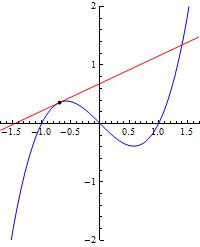### Concept to computation

The slope of the tangent line at $x=-0.7$ is about $0.47$. You could in fact calculate that value, to one decimal place anyway, by drawing a very careful picture of the graph of $y=x^3-x$, positioning the tangent line by eye and then measuring the run and rise with a ruler.

You can get a better estimate by looking at the secant lines that go through the point of tangency and one other nearby point. They are shown below for $x=-0.7.$ The other point in each case is $x=-0.7+h$ for $h=0.2,\, 0.1,\,0.06,\,0.02,\,0.01$ in that order.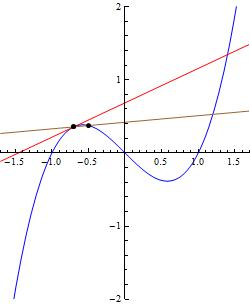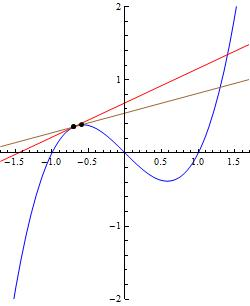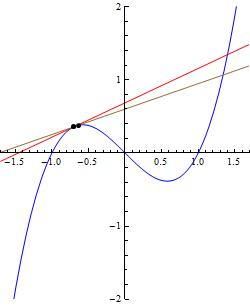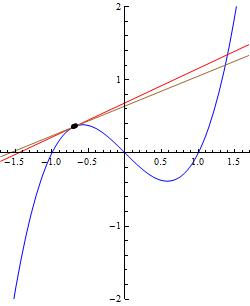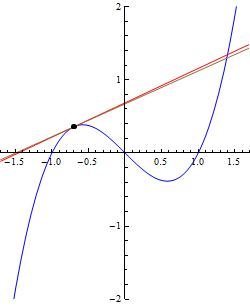### Computation to general formula

The slope of the secant line is $\frac{f(x+h)-f(x)}{x-(x-h)}=\frac{f(x+h)-f(x)}{h}$ when $h$ is small. For example, for $x=-0.7$ and $h=0.01$ the value is about $0.4491$, and when $h=0.001$ it is $0.467901$.

The trouble with getting the exact value of the slope, which is when $h=0$, is that it looks like you must divide by zero. However, a miracle occurs when $f(x)=x^3-x$: The formula for the slope simplifies to $3x^2+3xh+h^2-1$, so the limit when $h\to0$ is $3x^2-1$. So now we have a formula for the derivative function for $x^3-x$. If you have taken a little calculus, you may know that we can always get a formula for the derivative of a polynomial this way, and (with extra cleverness in some cases) for the derivatives of most other calculus-type functions.

This example shows how mathematicians (in this case in the seventeenth century) started from the naive or pictorial idea of the derivative as giving the slope of the tangent line and created calculations that allowed us to have a formula for the derivative of many functions.

There is more detail about this in my blog post The power of being naive, which has diagrams you can manipulate with your mouse.

#### Simplifying the formula for the slope

When $f(x)=x^3-x$, \begin{align} \dfrac{f(x+h)-f(x)}{h}&=\dfrac{(x+h)^3-x-h-x^3+x}{h}\\ &=\dfrac{x^3+3x^2h+3xh^2+h^3-x-h-x^3+x}{h}\\ &=\dfrac{3x^2h+3xh^2+h^3-h}{h}\\ &=3x^2+3xh+h^2-1\end{align}

Note that the terms that don't contain $h$ disappear in the third step. This always happens with any polynomial. Isn't that a miracle?

## Other examples